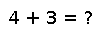# std

std(v[, region])

Compute the sample standard deviation of a vector or array `v`, optionally along dimensions in `region`. The algorithm returns an estimator of the generative distribution's standard deviation under the assumption that each entry of `v` is an IID drawn from that generative distribution. This computation is equivalent to calculating `sqrt(sum((v - mean(v)).^2) / (length(v) - 1))`. Note: Julia does not ignore `NaN` values in the computation. For applications requiring the handling of missing data, the `DataArray` package is recommended.

## Examples

Checking you are not a robot: